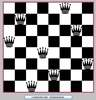Eight queens

1848 年

• 八皇后
#include <math.h>
#include <stdio.h>

#define MAX 8 // 宏定义皇后个数

typedef struct // 位置结构体
{
int x; // x 坐标
int y; // y 坐标
}Point;

int count = 0; // 统计方法的总数
Point queen[MAX + 2]; // 皇后数组

/**
@function:  将第index个皇后放在(x, y)的位置
@param:     x-->当前皇后所放的行
y-->当前皇后所放的列
index-->当前皇后的下标
@return:    void
*/
void putQueen(int x, int y, int index)
{
int i;
// 下标超出范围,返回
if (index > MAX)
{
return;
}
// 暂定当前皇后的位置
queen[index].x = x;
queen[index].y = y;

// 判断与前面放的皇后是否符合规则
for (i = 1; i<index; i++)
{
// 如果同行或同列有皇后,则返回
if (x == queen[i].x || y == queen[i].y)
{
return;
}
// 如果斜方向有皇后,则返回
if (abs(x - queen[i].x) == abs(y - queen[i].y))
{
return;
}
}
// 下标等于皇后个数,说明已经放完,输出当前情况
if (index == MAX)
{
printf("\n第 %d 种方法:\n", ++count);
for (i = 1; i <= MAX; i++)
{
printf("Queen %d: (%d, %d)\n", i, queen[i].x, queen[i].y);
}
return;
}
// 已经暂定index个皇后的位置,讨论第index+1个皇后的位置
for (i = 1; i <= MAX; i++)
{
putQueen(x + 1, i, index + 1);
// 讨论完一种情况后将index+1个皇后的的下标初始化
queen[index + 1].x = 0;
queen[index + 1].y = 0;
}
}

int main()
{
count = 0; // 初始化放法为0
putQueen(0, 0, 0); // 从第0个皇后开始
return 0;
}
展开全文递归...